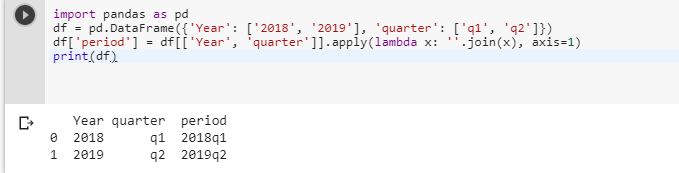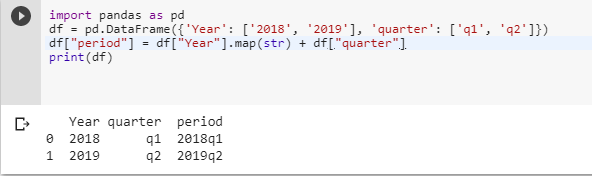2 views
in Python

I have a 20 x 4000 dataframe in python using pandas. Two of these columns are named Year and quarter. I'd like to create a variable called period that makes Year = 2000 and quarter= q2 into 2000q2

Can anyone help with that?

by (106k points)

There are many ways of combining two columns of text in a dataframe in pandas/python some of the important I am discussing here:-

The first method you can use is apply() function below is the code for the same:-

import pandas as pd

df = pd.DataFrame({'Year': ['2018', '2019'], 'quarter': ['q1', 'q2']})

df['period'] = df[['Year', 'quarter']].apply(lambda x: ''.join(x), axis=1)

print(df)The above method works by replacing df[['Year', 'quarter']] with any column slice of your dataframe, e.g. df.iloc[:,0:2].apply(lambda x: ''.join(x), axis=1).

The second way to solve this problem is to use map() function below is the piece of code that shows how to do it:-

import pandas as pd

df = pd.DataFrame({'Year': ['2018', '2019'], 'quarter': ['q1', 'q2']})

df["period"] = df["Year"].map(str) + df["quarter"]

print(df)